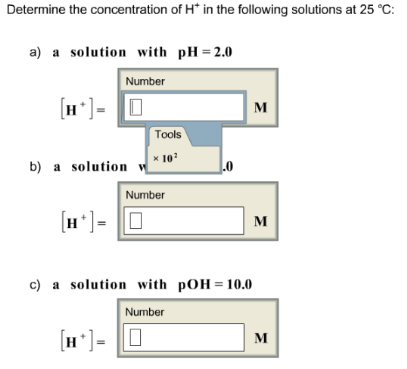# Problem: Determine the concentration of H+ in the following solutions at 25°C: a) a solution with pH = 2.0 [H+] =  b) a solution  [H+] =  c) a solution with pOH = 10.0 [H+] =

###### FREE Expert Solution
99% (489 ratings)###### Problem Details

Determine the concentration of H+ in the following solutions at 25°C:

a) a solution with pH = 2.0

[H+] =

b) a solution

[H+] =

c) a solution with pOH = 10.0

[H+] =